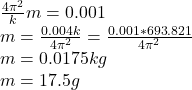## You hang different masses M from the lower end of a vertical spring and measure the period T for each value of M. You use Excel to plot T2 (

Question

You hang different masses M from the lower end of a vertical spring and measure the period T for each value of M. You use Excel to plot T2 (in s2) on the y-axis versus M (in kg) on the x-axis. The equation for the straight line that gives the best fit to your data is
y = 0.0569x + 0.00100.
A) What is the force constant k of the spring?
B) What is the mass of the spring?

in progress 0
5 months 2021-08-25T18:19:25+00:00 1 Answers 0 views 0

a)693.821N/m

b)17.5g

Explanation:

We the Period T we can find the constant k,

That is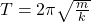squaring on both sides,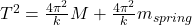where,

M=hanging mass, m = spring mass,

k =spring constant

T =time period

a) So for the equation we can compare, that is,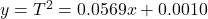the hanging mass M is x here, so comparing the equation we know that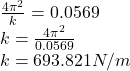b) In order to find the mass of the spring we make similar process, so comparing,